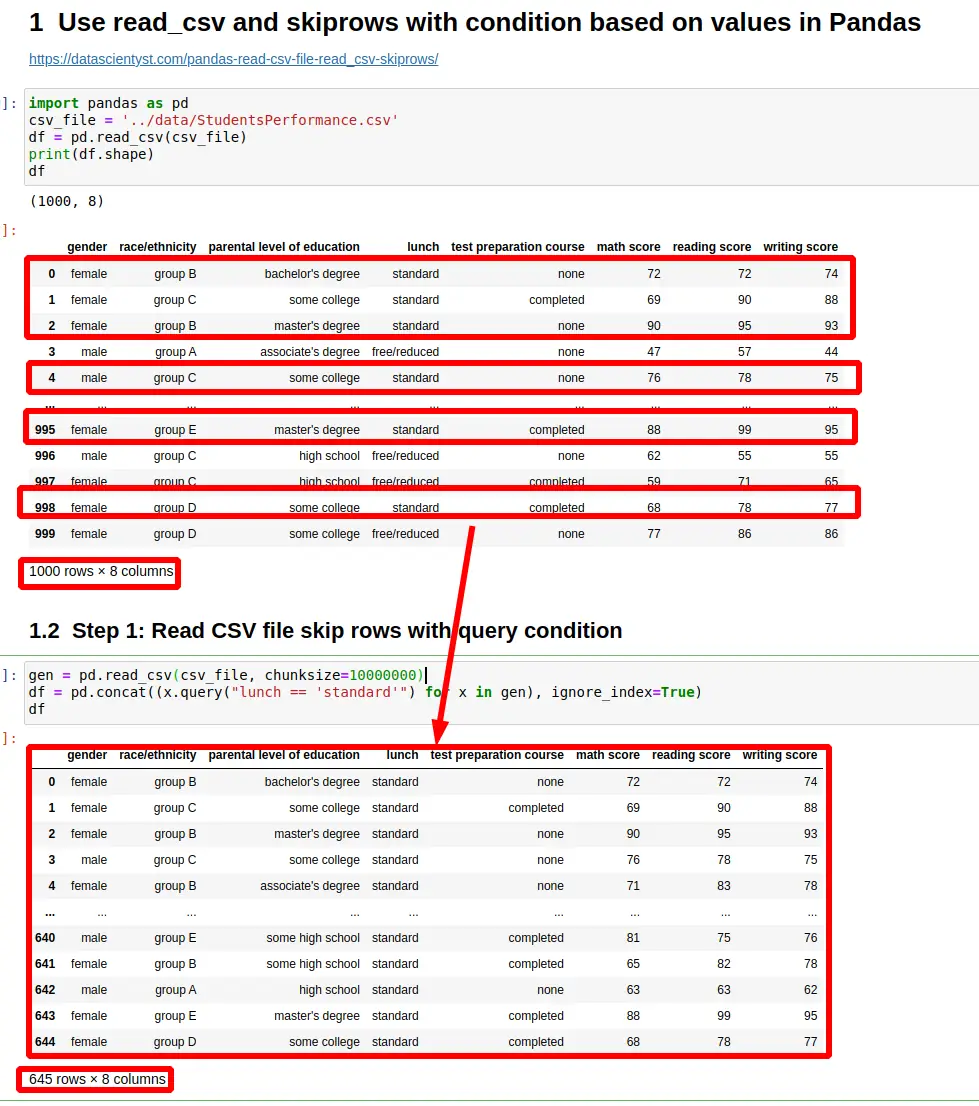In this tutorial, we'll look at how to read CSV files by `read_csv` and skip rows with a conditional statement in Pandas.

In addition, we'll also see how to optimise the reading performance of the `read_csv` method with Dask. This option is useful if you face memory issues using `read_csv`.

To start let's say that we have the following CSV file:

gender race/ethnicity math score reading score writing score
female group B 72 72 74
female group C 69 90 88
female group B 90 95 93
male group A 47 57 44
male group C 76 78 75
``````1000 rows × 8 columns
``````

## Step 1: Read CSV file skip rows with query condition in Pandas

By default Pandas `skiprows` parameter of method `read_csv` is supposed to filter rows based on row number and not the row content.

So the default behavior is:

``````pd.read_csv(csv_file, skiprows=5)
``````

The code above will result into:

``````995 rows × 8 columns
``````

But let's say that we would like to skip rows based on the condition on their content. This can be achieved by reading the CSV file in chunks with `chunksize`.

The results will be filtered by `query` condition:

``````gen = pd.read_csv(csv_file, chunksize=10000000)
df = pd.concat((x.query("lunch == 'standard'") for x in gen), ignore_index=True)
``````

result:

``````645 rows × 8 columns
``````

The above code will filter CSV rows based on column `lunch`. It will return only rows containing `standard` to the output.

## Step 2: Read CSV file with condition value higher than threshold

In this step we are going to compare the row value in the rows against integer value. If the value is equal or higher we will load the row in the CSV file.

Difference with the previous step is:

• the usage of `dtype` parameter which defines a schema for the columns read from the CSV file
• how to use query with column which contains space - `math score` - surrounded by `
``````schema={
"math score": int
}
df = pd.concat((x.query("`math score` >= 75") for x in gen), ignore_index=True)
``````

The code above will filter all rows which contain `math score` higher or equal to 75:

``````295 rows × 8 columns
``````

## Step 3: Read CSV file and post filter condition in Pandas

For small and medium CSV files it's fine to read the whole file and do a post filtering based on read values.

This can be achieved by:

``````df = pd.read_csv(csv_file)

df = df[df['lunch'] != 'standard']
``````

result:

``````355 rows × 8 columns
``````

So first we read the whole file. Next we are filtering the results based on one or multiple conditions.

Finally let's see how to read a CSV file with condition and optimised performance.

``````pip install dask
``````

Dask offers a lazy reader which can optimize performance of `read_csv`.

So first we can read the CSV file, then apply the filtering and finally to compute the results:

``````import dask.dataframe as dd

``````496 rows × 8 columns